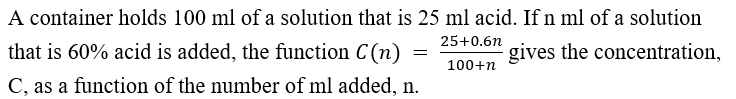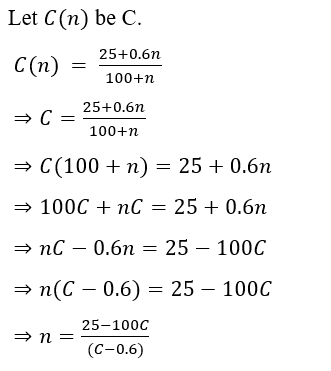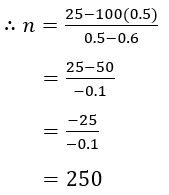# A container holds 100 ml of a solution that is 25 ml acid. If n ml of a solution that is 60% acid is added, the function C(n) = 25+0.6n/100+n  gives the concentration, C, as a function of the number of ml added, n. Express n as a function of C and determine the number of mL that need to be added to have a solution that is 50% acid. solve by pre-calc/ algebra methods please asap thank you

Question
30 views

A container holds 100 ml of a solution that is 25 ml acid. If n ml of a solution that is 60% acid is added, the function C(n) = 25+0.6n/100+n  gives the concentration, C, as a function of the number of ml added, n. Express n as a function of C and determine the number of mL that need to be added to have a solution that is 50% acid.

solve by pre-calc/ algebra methods please asap thank you

check_circle

star
star
star
star
star
1 Rating
Given:Solving for n:Now, evaluate this function at 50% which is C=0.5We can conclude that 250 mL added to have a solution that is 50% acid.

...

### Want to see the full answer?

See Solution

#### Want to see this answer and more?

Solutions are written by subject experts who are available 24/7. Questions are typically answered within 1 hour.*

See Solution
*Response times may vary by subject and question.
Tagged in

### Algebra